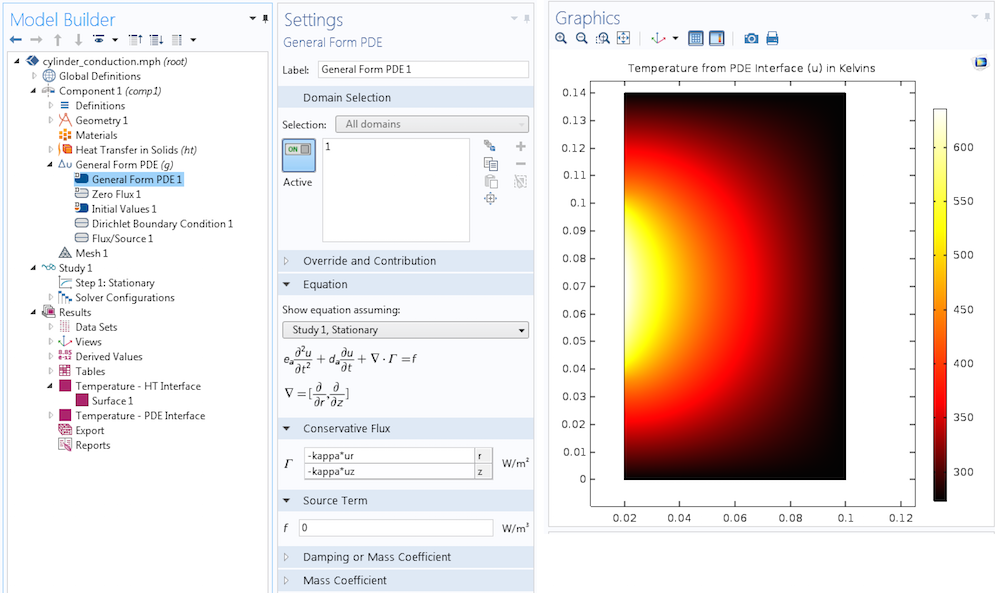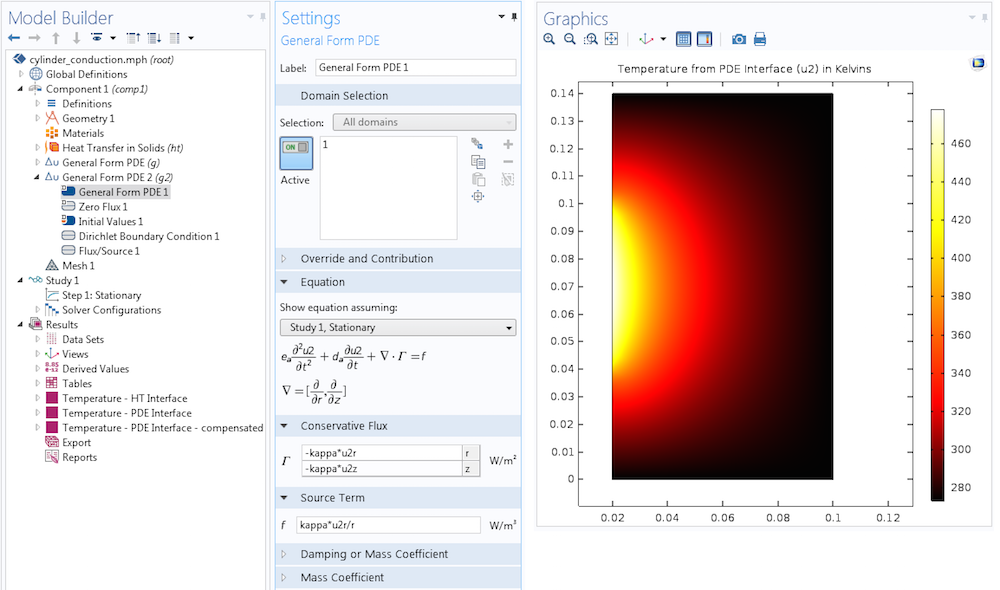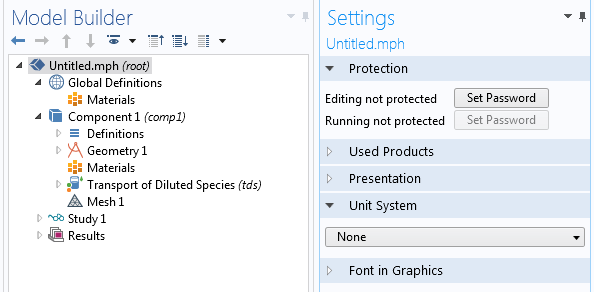# 基于方程的轴对称组件建模指南

2016年 10月 5日

### 一个轴对称的热传导问题

\rho C_p\mathbf{u}\cdot \nabla T + \nabla \cdot (-\kappa \nabla T)=Q,e_a\frac{\partial^2 u}{\partial t^2} + d_a\frac{\partial u}{\partial t} + \nabla \cdot \Gamma = f,\frac{\partial \Gamma_r}{\partial r} + \frac{\partial \Gamma_z}{\partial z}.

#### 守恒定律

\rho C_p\frac{\partial T}{\partial t}+\rho C_p\mathbf{u}\cdot \nabla T + \nabla \cdot (-\kappa \nabla T)=Q,

#### 坐标系

\nabla T = \frac{\partial T}{\partial x}\mathbf{e}_x + \frac{\partial T}{\partial y}\mathbf{e}_y+\frac{\partial T}{\partial z}\mathbf{e}_z,

\nabla T = \frac{\partial T}{\partial r}\mathbf{e}_r + \frac{1}{r}\frac{\partial T}{\partial \phi}\mathbf{e}_{\phi}+\frac{\partial T}{\partial z}\mathbf{e}_z.

\nabla \cdot (\kappa \nabla T) = \frac{\partial }{\partial x}(\kappa \frac{\partial T}{\partial x}) + \frac{\partial }{\partial y}(\kappa \frac{\partial T}{\partial y}) + \frac{\partial }{\partial z}(\kappa \frac{\partial T}{\partial z}) = \frac{1}{r}\frac{\partial }{\partial r}(r \kappa \frac{\partial T}{\partial r}) + \frac{1}{r}\frac{\partial }{\partial \phi}( \frac{\kappa}{r}\frac{\partial T}{\partial \phi})+\frac{\partial }{\partial z}(\kappa \frac{\partial T}{\partial z}).

### 比较轴对称组件中的物理场接口与偏微分方程接口

\nabla \cdot \Gamma = \frac{1}{r}\frac{\partial (r \Gamma)}{\partial r} + \frac{1}{r}\frac{\partial \Gamma_{\phi}}{\partial \phi}+\frac{\partial \Gamma_z}{\partial z}.

(1)

\nabla \cdot \Gamma = \frac{1}{r}\frac{\partial (r \Gamma_r)}{\partial r} +\frac{\partial \Gamma_z}{\partial z} = \frac{\partial \Gamma_r}{\partial r} + \frac{\partial \Gamma_z}{\partial z}+\frac{\Gamma_r}{r}.

### 轴对称热传导问题的解决方案

\nabla \cdot \Gamma = f,

\frac{\partial \Gamma_r}{\partial r} + \frac{\partial \Gamma_z}{\partial z}+\frac{\Gamma_r}{r}=f.

\frac{\partial \Gamma_r}{\partial r} + \frac{\partial \Gamma_z}{\partial z}=-\frac{\Gamma_r}{r}+f.### 将物理场接口用作偏微分方程模板### 关于基于方程的轴对称组件建模的总结性思考

• Rutherford Aris, Vectors, Tensors, and the Basic Equations of Fluid Mechanics, Dover Publications, Inc., 1989
• Pavel Grinfeld, Introduction to Tensor Analysis and the Calculus of Moving Surfaces, Springer Science+Business Media, 2013
• Mikhail Itskov, Tensor Algebra and Tensor Analysis for Engineers: with Applications to Continuum Mechanics, Springer-Verlag, 2013

#### 评论 (9)

##### 留言##### 飞龙 岳
2019-03-25##### Haili Wang
2019-04-15

COMSOL的偏导可以通过d()算子来书写，例如d(u,phi)表示的就是u在phi方向上的一阶偏导，那么二阶偏导的表达式可以写成d(d(u,phi),phi) 。##### 嘉林 张
2019-12-04##### 屹磊 金
2019-12-05##### 嘉林 张
2019-12-05##### 屹磊 金
2019-12-23##### 嘉林 张
2019-12-27##### 凯明 王
2019-12-20##### Shengyu Jia
2020-02-04

1）您提到的“将物理场接口用作偏微分方程模板”，具体是什么意思呢？怎样操作可以使得我仍使用这些接口中的微分算子的张量意义呢？
2）如何使用PDE写旋度？